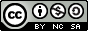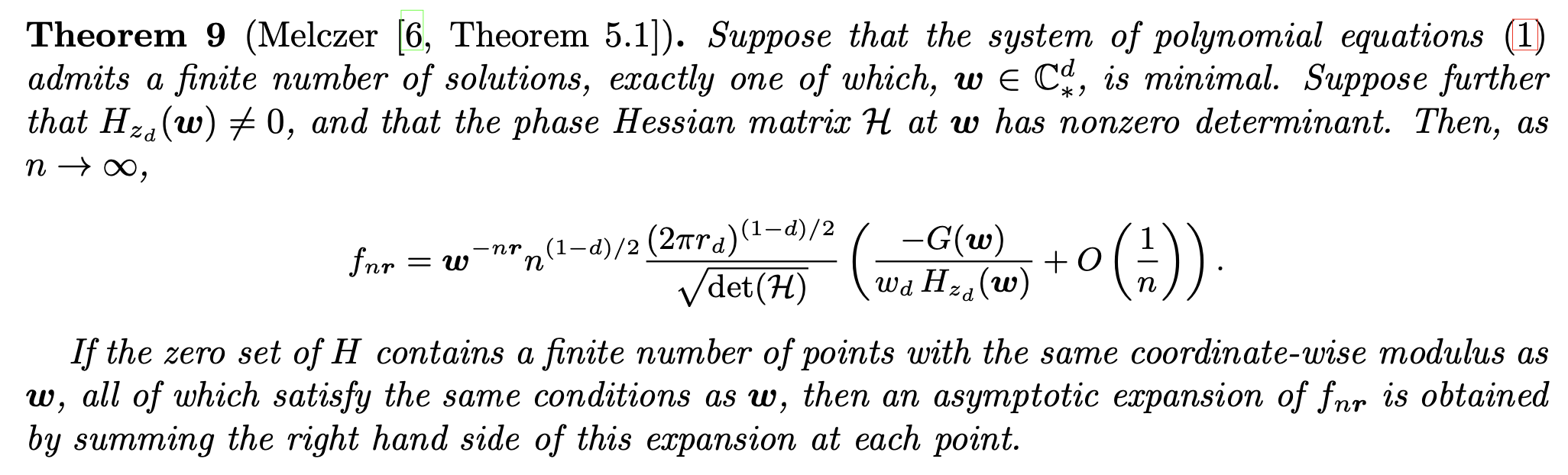ACSV in SageMath
Benjamin Hackl • July 21, 2023

## Rigorous analytic combinatorics in several variables in SageMath

Joint work with Andrew Luo, Stephen Melczer, Jesse Selover, Elaine Wong
##### (Interactive) Software Demo • FPSAC 2023 @ Davis, CAThis work is licensed under a Creative Commons Attribution-NonCommercial-ShareAlike 4.0 International License.### Overview: sage_acsv

• Input: multivariate, rational, combinatorial generating function $F(\mathbf{z}) = \sum_{i_1, i_2, ..., i_d \geq 0} f_{i_1, ..., i_d} z_1^{i_1} \cdots z_d^{i_d},$ and direction vector $\mathbf{r} = (r_1, ..., r_d) \in \mathbb{N}^d$
• Output: asymptotic expansion for diagonal $f_{n \mathbf{r}}$ for $n\to\infty$

### How does it work?

• $F(\mathbf{z}) = G(\mathbf{z})/H(\mathbf{z})$, with $G$ and $H$ (multivariate) polynomials
• Multivariate CIF: $f_{n\mathbf{r}} = \frac{1}{(2\pi i)^d} \int_{\mathcal{C}} \frac{G(\mathbf{z})}{H(\mathbf{z})} \frac{d\mathbf{z}}{\mathbf{z}^{n \mathbf{r} + 1}}$
• Singularity set is algebraic variety, has infinitely many "minimal" points...
• Under "mild" conditions on $H$ ($H(0)\neq 0$; $H$ and all partial derivatives do not vanish simultaneously; finitely many smooth critical points, at least one of which is minimal; phase Hessian non-singular at these points) special ("critical") minimal points determine asymptotics cf. [Pemantle-Wilson], [Baryshnikov-Pemantle] ...### Algorithmic Approach

• Find critical points $\mathbf{w} \in \mathbb{C}_{*}^d$ (via $H(\mathbf{w}) = 0$ and $\mathbf{0}\neq \operatorname{diag}(\mathbf{w}) \nabla H(\mathbf{w}) \| \mathbf{r}$)
• Check which critical points are minimal

Use all-in-one strategyvia [Melczer-Salvy]:

• Consider system $H(t \mathbf{w}) = 0$, $\operatorname{diag}(w) \nabla H(\mathbf{w}) = \lambda \mathbf{r}$, encode solutions with Kronecker representationlinear form $u - \kappa \mathbf{z} = 0$, polynomials $P$, $Q_1$, ..., $Q_d$ s.t. $Q_j(u)/P'(u) = z_j$ determine components of solution vector for $u$ iterating through roots of $P$ -- bounded coefficients / degrees!
• Check positive ($\sim$ Pringsheim!) solutions for $t = 1$
• Use solutions for $t\in (0,1)$ to eliminate non-minimal $\mathbf{w}$